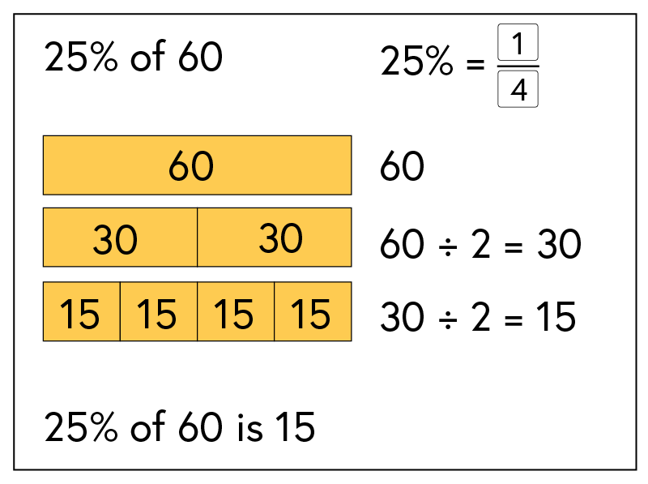3.10

# Finding a percentage of an amount

If we want to find 25% of 60, this is the same as finding a quarter of 60. Finding a quarter is relatively simple: we halve and then halve again.

Half of 60 is 30. Half of 30 is 15.

25% of 60 = 15.